CIESEmath Activities List (Version 2.1)
Source: Original Version 1.0 developed at CIESE -
Center for Innovation in Engineering & Science Education (2007
)
Last revision: 2/10/21Road Sign Problem Is there anything wrong with the sign? If so, what's the problem? Please explain.Pool paths Here is an unusual pool table. Your Challenge: See if you can come up with a way to predict (1) what the number of touches will be and (2) which corner the ball will end up.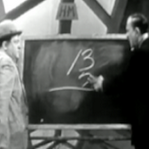13 x 7 = 28 Watch Abbott and Costello do some mathematical hocus-pocus with multiplying 13 x 7 and getting an answer of 28. Explain how Costello came up with an answer of 28.Birthday problem What is the probability that in a room of 25 people that 2 people share the same birthday? Use the applet to determine the probability experimentally.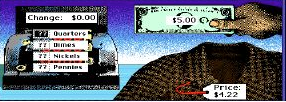Boutique Shop In this shop the student plays the role of a store clerk who sells clothes. In the image, the student is given \$5.00 for an item that costs \$4.22.  What are the different ways you make this change?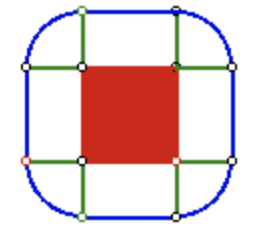String Around the Earth Problem Seymour Papert writes about a classic problem from the perspective of trying to understand the math behind our intuition that goes awry.Tris or Untris You will see 9 line segments (or straws) of various lengths. Make as many unique triangles using these segments. Determine when any three segments make a triangle.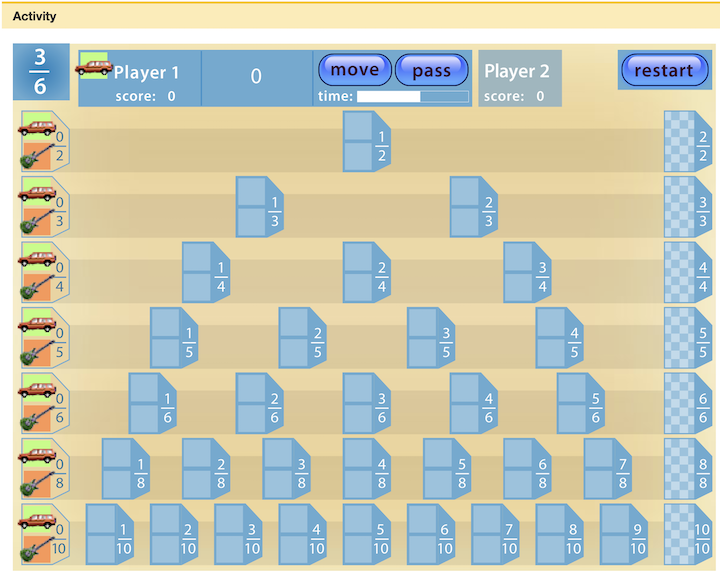Fraction Tracks In this 2-player game, students take turns racing to the end of each fraction line by moving one or more of their markers to a given fraction value.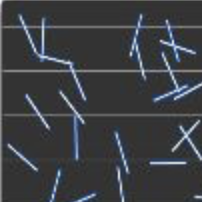Buffon's Needle Surprise The challenge here is to see if the students can make a prediction about the likelihood of toothpicks landing on lines when dropped randomly. And it's connection to π.Jinx Puzzle Pick a number, Add 11, multiply by 6, subtract 3, divide by 3, Add 5, Divide by 2, Subtract the original number. What did you get? Why is this called the Jinx puzzle?Family Fractions Today you are going to be a fraction that lives in Number Town. There are 5 families in the room. Your task is to find your family members.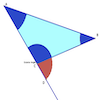Exterior Angle of a Triangle What is the relationship between the exterior angle and the non-adjacent angles of a triangle?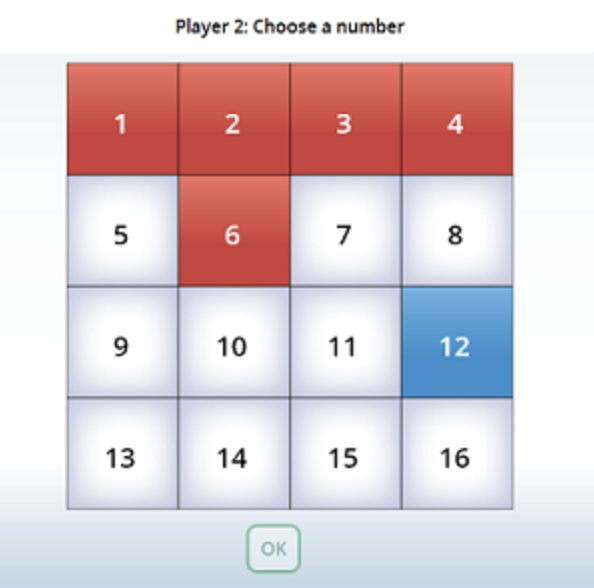The Factor Game Split the class into 2 groups and assign a captain to each group. On the white board tape 16 3x5 cards numbered from 1 to 16. Players take turns picking cards.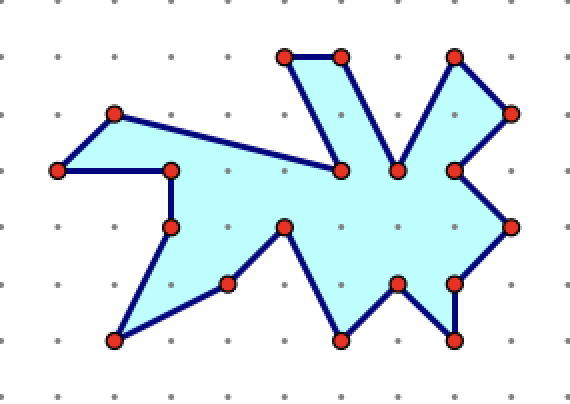Discover an equation that determines area by counting the number of boundary points (B) and the inside the polygon points (I).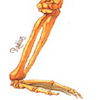Estimating Heights In a letter a professor asks your class: Is there a way to predict the height of a person by just knowing the length of the radius bone? Your students will need to to measure to find out.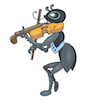Can predict the temperature by knowing a cricket's chirps per minute? (Chirps will greet you.)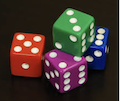Dice Races In this activity you will use a Scratch Applet to roll two cubes many times. The object is to see if you can predict which number will come up most often and win the race.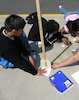Measure the Earth In measuring the circumference of earth, would you need to walk around the world to find out? Do you think you can do it with just a meter stick in one location? Try this project to find out!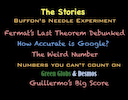Math Stories with a Surprise Twist My last CLIME presentation. It runs 20 minutes and 57 seconds.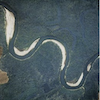The Shrinking Mississippi River The goal of the lesson is to get students to understand and appreciate how graphs can tell meaningful stories.